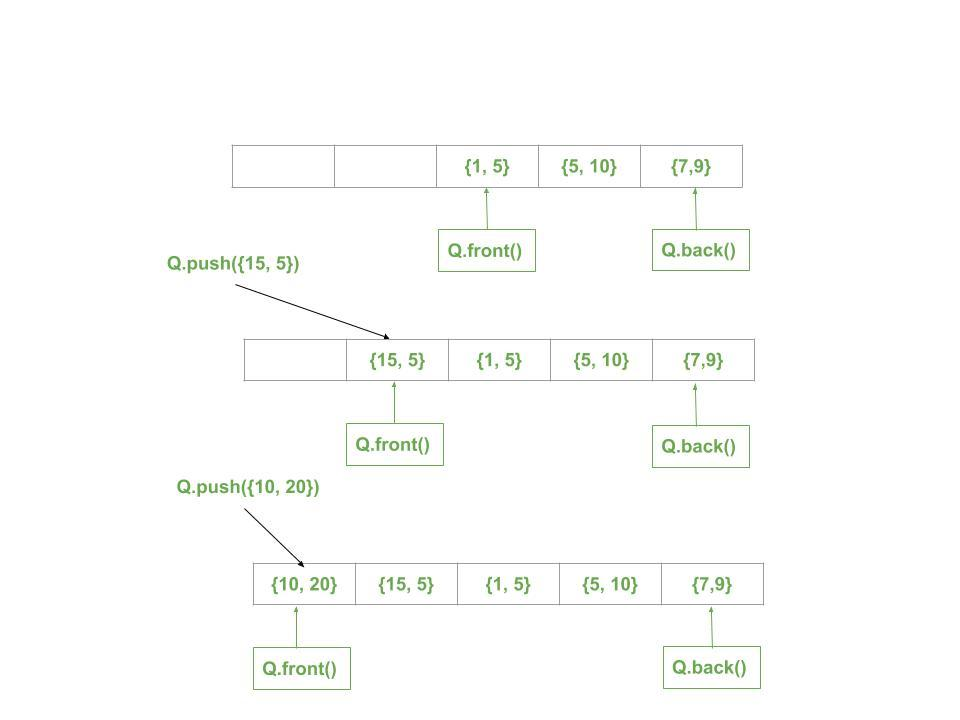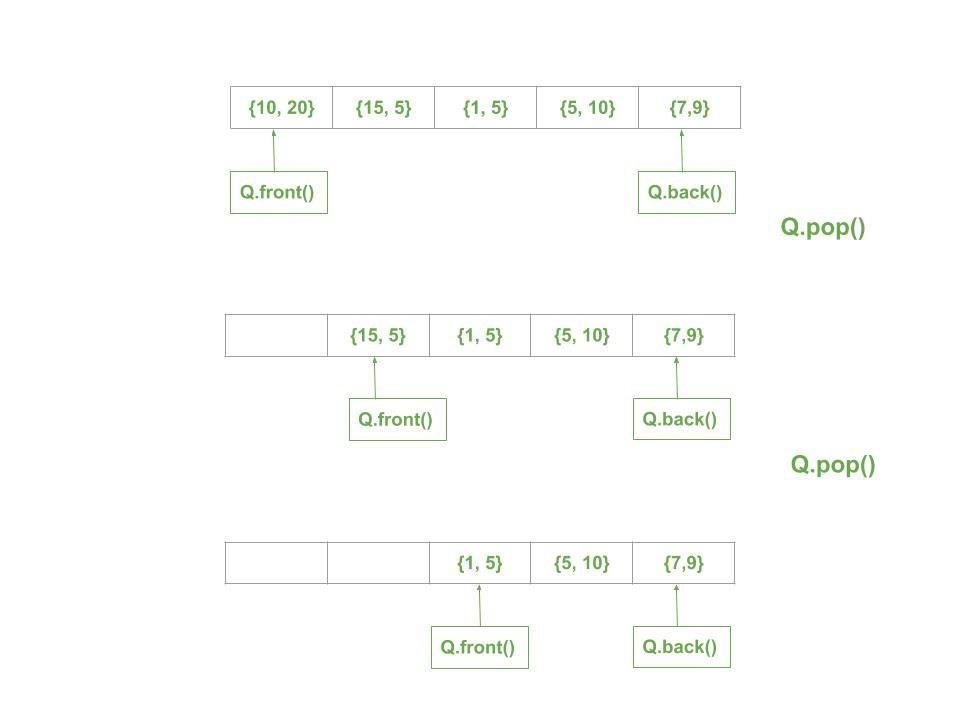# Queue of Pairs in C++ STL with Examples

Queue in STL are a type of container adaptors which operate in a first in first out (FIFO) type of arrangement where elements are inserted at the back (end) and are deleted from the front. Queue of pair can be very efficient in designing complex data structures. The first element is referenced as ‘first’ and the second element as ‘second’ and the order is fixed (first, second).

Syntax:

Queue<pair<datatype, datatype>> queue_of_pair;

Below are the images to show the working of Queue of Pairs:Below is an example to show the Queue of Pairs:

 `// C++ program to demonstrate ` `// the working of STL queue of pairs ` ` `  `#include ` `using` `namespace` `std; ` ` `  `// Print the current pair ` `void` `printPair(pair<``int``, ``int``> p) ` `{ ` `    ``// Gives first element from queue pair ` `    ``int` `f = p.first; ` ` `  `    ``// Gives second element from queue pair ` `    ``int` `s = p.second; ` ` `  `    ``cout << ``"("` `<< f << ``", "` `<< s << ``") "``; ` `} ` ` `  `// Print the Queue of Pairs ` `void` `showQueue(queue > gq) ` `{ ` `    ``// Print element untill the ` `    ``// queue is not empty ` `    ``while` `(!gq.empty()) { ` `        ``printPair(gq.front()); ` `        ``gq.pop(); ` `    ``} ` ` `  `    ``cout << ``'\n'``; ` `} ` ` `  `// Driver code ` `int` `main() ` `{ ` `    ``queue > gq; ` ` `  `    ``// Pushing elements inside ` `    ``// the queue container ` `    ``gq.push({ 10, 20 }); ` `    ``gq.push({ 15, 5 }); ` `    ``gq.push({ 1, 5 }); ` `    ``gq.push({ 5, 10 }); ` `    ``gq.push({ 7, 9 }); ` ` `  `    ``cout << ``"Queue of Pairs: "``; ` `    ``showQueue(gq); ` ` `  `    ``// Prints size of queue ` `    ``cout ` `        ``<< ``"\nSize of Queue of Pairs: "` `        ``<< gq.size(); ` ` `  `    ``// Prints first element ` `    ``// of queue container ` `    ``cout << ``"\nFront of Queue of Pairs: "``; ` `    ``printPair(gq.front()); ` ` `  `    ``// Prints last element ` `    ``// of queue container ` `    ``cout << ``"\nBack of Queue of Pairs: "``; ` `    ``printPair(gq.back()); ` ` `  `    ``cout << ``"\n\nRemoving the Front pair\n"``; ` `    ``gq.pop(); ` `    ``cout << ``"Current Queue of Pairs: "``; ` `    ``showQueue(gq); ` ` `  `    ``return` `0; ` `} `

Output:

```Queue of Pairs: (10, 20) (15, 5) (1, 5) (5, 10) (7, 9)

Size of Queue of Pairs: 5
Front of Queue of Pairs: (10, 20)
Back of Queue of Pairs: (7, 9)

Removing the Front pair
Current Queue of Pairs: (15, 5) (1, 5) (5, 10) (7, 9)
```

Attention reader! Don’t stop learning now. Get hold of all the important DSA concepts with the DSA Self Paced Course at a student-friendly price and become industry ready.

My Personal Notes arrow_drop_upCheck out this Author's contributed articles.

If you like GeeksforGeeks and would like to contribute, you can also write an article using contribute.geeksforgeeks.org or mail your article to contribute@geeksforgeeks.org. See your article appearing on the GeeksforGeeks main page and help other Geeks.

Please Improve this article if you find anything incorrect by clicking on the "Improve Article" button below.